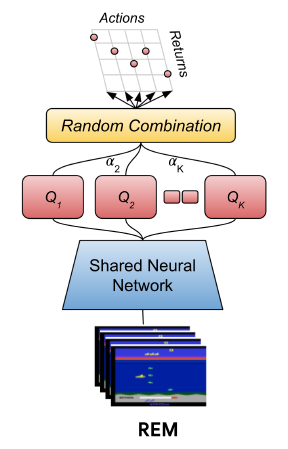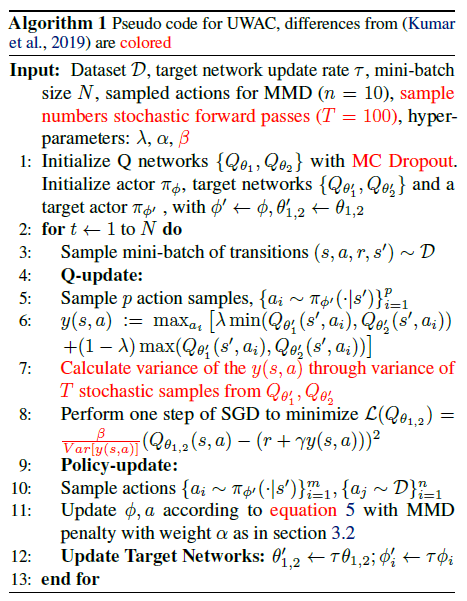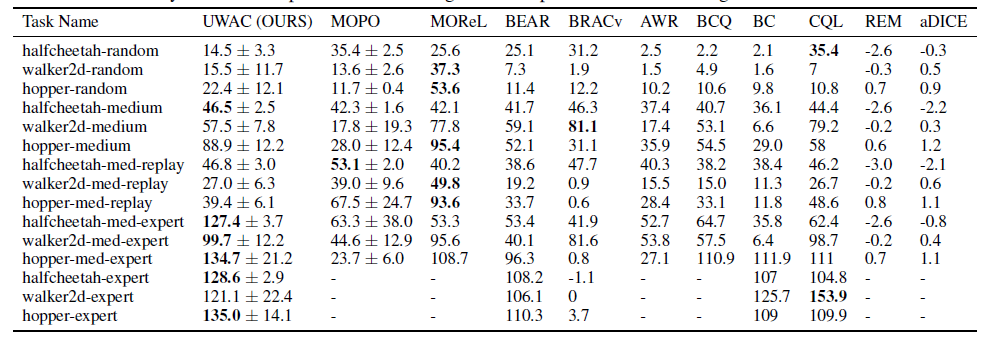# 离线强化学习中的不确定性

• Aleatoric uncertainty，也就是随机不确定性，也可以称为数据不确定性。它来源于训练数据本身的噪声，是随机而且固定的，是无法通过增加数据减小的。
• Epistemic uncertainty，也就是认知不确定性，也可以称为模型不确定性。这种主要是由于模型预测不好导致的，可以通过增加数据去解决这个问题。

• 在Model-based的强化学习中，使用的方式是（待更新）
• 在Model-free的强化学习中，主要是用集成的方式和MC Dropout的方式来对Q函数的不确定性进行建模。

$$$\pi_{k+1} = \argmax_{\pi} \mathbb{E}_{a\sim \mathcal{D}}[\mathbb{E}_{a\sim \pi(a|s)}[\mathbb{E}_{Q^\pi_{k+1}\sim \mathcal{P}_\mathcal{D}(Q^\pi)}[Q^\pi_{k+1}(s,a)] - \alpha Unc(\mathcal{P}_\mathcal{D}(Q^\pi))]]$$$

## 集成方法

$$$Q(s,a)=\sum_{i=1}^N \alpha_iQ_{\phi_i}(s,a), \sum_{i=1}^N\alpha_i=1$$$## Monte Carlo Dropout方法

$$$p(\theta|X, Y) = \frac{P(Y|X,\theta)P(\theta)}{P(Y|X)}$$$

$$$\mathbb{V}[Q(s,a)] \approx \sigma^2 + \frac{1}{T}\sum_{i=1}^T\hat{Q}_t(s,a)^T\hat{Q}_t(s,a) - \mathbb{E}[\hat{Q}(s,a)]^T\mathbb{E}[\hat{Q}(s,a)]$$$

$$$\pi'(a|s) = \frac{\beta}{\mathbb{V}[Q_0^{\pi'}(s,a)]}\pi(a|s)/Z(s) \\ Z(s) = \int_a \frac{\beta}{\mathbb{V}[Q_0^{\pi'}(s,a)]}\pi(a|s) da$$$

Critic的损失函数：

$$$\mathcal{L}(Q_\theta)=\mathbb{E}_{(s'|s,a)\sim D}\mathbb{E}_{a\sim \pi}\Big[\frac{\beta}{\mathbb{V}[Q_0^{\pi'}(s,a)]}*Err(s,a,s',a')^2\Big]$$$

$$$\mathcal{L}(\pi)=-\mathbb{E}_{a\sim \pi}\Big[\frac{\beta}{\mathbb{V}[Q_0^{\pi'}(s,a)]}Q_\theta(s,a)\Big]$$$

UWAC是在基线算法BEAR$^{}$上进行改进的，伪代码如下：UWAC的做法是很直观的，并且对原基线算法的改动很小（红色标记）。UWAC用dropout的方式得到多个Q值。如果本身我们能对这个样本进行准确估计的时候，这个方差就会比较小，从而使得策略更偏向于选择这个样本对应的动作。如果不能准确估计则相反。## 参考文献

 Kendall, Alex, and Yarin Gal. "What Uncertainties Do We Need in Bayesian Deep Learning for Computer Vision?." Advances in neural information processing systems (NeurIPS), 2017.

 Agarwal, R., Schuurmans, D., and Norouzi, M. An optimistic perspective on offline reinforcement learning. In International Conference on Machine Learning, pp. 104–114. PMLR, 2020.

 Wu, Yue, et al. "Uncertainty Weighted Actor-Critic for Offline Reinforcement Learning." International Conference on Machine Learning (ICML). PMLR, 2021.

 Gal, Y. and Ghahramani, Z. Dropout as a bayesian approximation: Representing model uncertainty in deep learning. In international conference on machine learning (ICML), pp.1050–1059, 2016.

 Kumar, A., Fu, J., Soh, M., Tucker, G., and Levine, S. Stabilizing off-policy q-learning via bootstrapping error reduction. In Advances in Neural Information Processing Systems (NeurIPS), 2019.